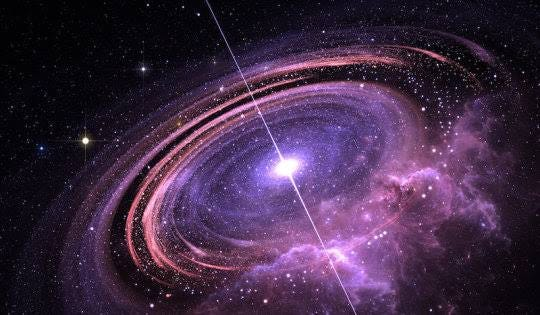# Predicting a Pulsar Star using different Machine Learning Algorithms

Source: Deep Learning on Medium# INTRODUCTION

Pulsars are spherical, compact objects that are about the size of a large city but contain more mass than the sun. Scientists are using pulsars to study extreme states of matter, search for planets beyond Earth’s solar system and measure cosmic distances. Pulsars also could help scientists find gravitational waves, which could point the way to energetic cosmic events like collisions between super massive black holes. Discovered in 1967, pulsars are fascinating members of the cosmic community.

Pulsars radiate two steady, narrow beams of light in opposite directions. Although the light from the beam is steady, pulsars appear to flicker because they also spin. It’s the same reason a lighthouse appears to blink when seen by a sailor on the ocean: As the pulsar rotates, the beam of light may sweep across the Earth, then swing out of view, then swing back around again. To an astronomer on the ground, the light goes in and out of view, giving the impression that the pulsar is blinking on and off. The reason a pulsar’s light beam spins around like a lighthouse beam is that the pulsar’s beam of light is typically not aligned with the pulsar’s axis of rotation.

Each pulsar produces a slightly different emission pattern, which varies slightly with each rotation . Thus a potential signal detection known as a ‘candidate’, is averaged over many rotations of the pulsar, as determined by the length of an observation. In the absence of additional info, each candidate could potentially describe a real pulsar. However in practice almost all detection are caused by radio frequency interference (RFI) and noise, making legitimate signals hard to find.

Machine learning tools are now being used to automatically label pulsar candidates to facilitate rapid analysis. Classification systems in particular are being widely adopted, which treat the candidate data sets as binary classification problems.

This blog takes up different Machine Learning classification algorithms to depict the prediction of a pulsar star. The dataset used for building the model is available at www.kaggle.com .

# USING VARIOUS MACHINE LEARNING ALGORITHMS

We will be using Supervised Learning in our model to reach the desired output. In general, supervised learning occurs when a system is given input and output variables with the intentions of learning how they are mapped together, or related. The goal is to produce an accurate enough mapping function that when new input is given, the algorithm can predict the output. This is an iterative process, and each time the algorithm makes a prediction, it is corrected or given feedback until it achieves an acceptable level of performance.

Our problem calls for deploying Classification algorithms. Brief;y, Classification either predicts categorical class labels or classifies data (construct a model) based on the training set and the values (class labels) in classifying attributes and uses it in classifying new data. There are a number of classification models. Classification models include logistic regression, decision tree, random forest, gradient-boosted tree, multi-layer perceptron, one-vs-rest, and Naive Bayes.

# Dataset

Let us take a look at the dataset that we are using for running various Classification algorithms.

Each row lists the variables first, and the class label is the final entry. The class labels used are 0 (negative) and 1 (positive). Each candidate is described by 8 continuous variables, and a single class variable. The first four are simple statistics obtained from the integrated pulse profile (folded profile). This is an array of continuous variables that describe a longitude-resolved version of the signal that has been averaged in both time and frequency . The remaining four variables are similarly obtained from the DM-SNR curve . These are summarized below:

1. Mean of the integrated profile. (DECIMAL)
2. Standard deviation of the integrated profile. (DECIMAL)
3. Excess kurtosis of the integrated profile. (DECIMAL)
4. Skewness of the integrated profile. (DECIMAL)
5. Mean of the DM-SNR curve. (DECIMAL)
6. Standard deviation of the DM-SNR curve. (DECIMAL)
7. Excess kurtosis of the DM-SNR curve. (DECIMAL)
8. Skewness of the DM-SNR curve. (DECIMAL)
9. Class (INTEGER)

Next, let us import the necessary libraries required for building our model(s).